# WA Analysis Filter Bank VI

LabVIEW 2014 Advanced Signal Processing Toolkit Help

Edition Date: June 2014

Part Number: 372656C-01

»View Product InfoDownload Help (Windows Only)

Owning Palette: Filter Banks VIs

Computes the single-level discrete wavelet transform (DWT) using a two-channel analysis filter bank. This VI decomposes the signal into the low-frequency subband (approx coef) and the high-frequency subband (detail coef). Both subbands have half the sampling rate of signal. Wire data to the signal input to determine the polymorphic instance to use or manually select the instance.

Examples

Use the pull-down menu to select an instance of this VI.

 Select an instance WA 1D Analysis Filter Bank (Waveform)WA 1D Analysis Filter Bank (Array)WA 2D Analysis Filter Bank

## WA 1D Analysis Filter Bank (Waveform)extension specifies the method to use to pad data at the borders of the input signal. The extension length is equal to the length of the wavelet filters. When you select the extension method, make the transition between the input signal and the padded data as smooth as possible because a smooth transition generates fewer large detail coefficients and enhances the efficiency of the signal representation.

 0 Zero padding—Uses zeros to pad the input data. Watch for abrupt transitions between the padded zeros and the input data, which causes large artifacts near the transition. 1 Symmetric (default)—Uses replications of the input data to pad the data, except that this VI left-flips the block at the input and right-flips the block at the end. 2 Periodic—Adds a replication of the input data block before and another replication after the input data block to pad the data.signal specifies the input signal.error in describes error conditions that occur before this node runs. This input provides standard error in functionality.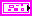analysis filters specifies the coefficients of the lowpass analysis filters and the highpass analysis filters. You can use the WA Wavelet Filter VI to obtain the filters of commonly used wavelets. You can use the Wavelet Design Express VI to design the analysis filters and the corresponding synthesis filters.lowpass specifies the coefficients of the lowpass analysis filter, which this VI uses to compute the approximation coefficients.highpass specifies the coefficients of the highpass analysis filter, which this VI uses to compute the detail coefficients.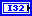shift specifies the index where decimation starts after this VI filters the signal. shift must be 0 or 1.approx coef returns the approximation coefficients, which are the output of the lowpass analysis filter followed by a decimation factor of 2.detail coef returns the detail coefficients, which are the output of the highpass analysis filter followed by a decimation factor of 2.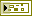error out contains error information. This output provides standard error out functionality.

## WA 1D Analysis Filter Bank (Array)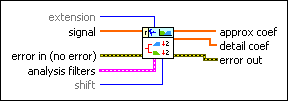extension specifies the method to use to pad data at the borders of the input signal. The extension length is equal to the length of the wavelet filters. When you select the extension method, make the transition between the input signal and the padded data as smooth as possible because a smooth transition generates fewer large detail coefficients and enhances the efficiency of the signal representation.

 0 Zero padding—Uses zeros to pad the input data. Watch for abrupt transitions between the padded zeros and the input data, which causes large artifacts near the transition. 1 Symmetric (default)—Uses replications of the input data to pad the data, except that this VI left-flips the block at the input and right-flips the block at the end. 2 Periodic—Adds a replication of the input data block before and another replication after the input data block to pad the data.signal specifies the input signal.error in describes error conditions that occur before this node runs. This input provides standard error in functionality.analysis filters specifies the coefficients of the lowpass analysis filters and the highpass analysis filters. You can use the WA Wavelet Filter VI to obtain the filters of commonly used wavelets. You can use the Wavelet Design Express VI to design the analysis filters and the corresponding synthesis filters.lowpass specifies the coefficients of the lowpass analysis filter, which this VI uses to compute the approximation coefficients.highpass specifies the coefficients of the highpass analysis filter, which this VI uses to compute the detail coefficients.shift specifies the index where decimation starts after this VI filters the signal. shift must be 0 or 1.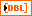approx coef returns the approximation coefficients, which are the output of the lowpass analysis filter followed by a decimation factor of 2.detail coef returns the detail coefficients, which are the output of the highpass analysis filter followed by a decimation with a factor of 2.error out contains error information. This output provides standard error out functionality.

## WA 2D Analysis Filter Bankextension specifies the method to use to pad data at the borders of the input signal. The extension length is equal to the length of the wavelet filters. When you select the extension method, make the transition between the input signal and the padded data as smooth as possible because a smooth transition generates fewer large detail coefficients and enhances the efficiency of the signal representation.

 0 Zero padding—Uses zeros to pad the input data. Watch for abrupt transitions between the padded zeros and the input data, which causes large artifacts near the transition. 1 Symmetric (default)—Uses replications of the input data to pad the data, except that this VI left-flips the block at the input and right-flips the block at the end. 2 Periodic—Adds a replication of the input data block before and another replication after the input data block to pad the data.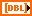signal specifies the 2D input signal.error in describes error conditions that occur before this node runs. This input provides standard error in functionality.analysis filters specifies the coefficients of the lowpass analysis filters and the highpass analysis filters. You can use the WA Wavelet Filter VI to obtain the filters of commonly used wavelets. You can use the Wavelet Design Express VI to design the analysis filters and the corresponding synthesis filters.lowpass specifies the coefficients of the lowpass analysis filter, which this VI uses to compute the approximation coefficients.highpass specifies the coefficients of the highpass analysis filter, which this VI uses to compute the detail coefficients.shift specifies the index where decimation starts after this VI filters the signal. shift must be 0 or 1.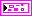DWT coef returns the approximation coefficients and the detail coefficients from the 2D single-level discrete wavelet transform (DWT).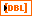low_low returns the approximation coefficients from the lowpass analysis filtering on each row and each column. The low_low coefficients are a low-resolution approximation of the original 2D signal.low_high returns the detail coefficients from the lowpass analysis filtering on each row and the highpass analysis filtering on each column. The high-frequency signal along the column direction influences the low_high coefficients.high_low returns the detail coefficients from the highpass analysis filtering on each row and the lowpass analysis filtering on each column. The high-frequency signal along the row direction influences the high_low coefficients.high_high returns the detail coefficients from the highpass analysis filtering on each row and each column. The high-frequency signal along the diagonal direction influences the high_high coefficients.error out contains error information. This output provides standard error out functionality.

## Examples

Refer to the following VIs for examples of using the WA Analysis Filter Bank VI:

• Filter Banks - 1D VI: labview\examples\Wavelet Analysis\WAGettingStarted
• Filter Banks - 2D VI: labview\examples\Wavelet Analysis\WAGettingStarted
• Wavelet Order Selection VI: labview\examples\Wavelet Analysis\WAGettingStarted